# How to Calculate Profit in Excel

Do you know how to calculate profit percentage? Whatever small business you run, you should know some basic bookkeeping things such as how to calculate profit and loss in Excel. If you know to keep track of your business finances, you will have a more clear picture of what should be your next steps to take.

As most of you know, Microsoft Excel can be a very powerful bookkeeping tool for anyone running a small business of their own. It enables users to easily and effectively keep track of money coming in and leaving the company with easy-to-follow spreadsheets and formulas that are very simple to use when calculating profits and expenses. Here is a quick guide on how to calculate profit by putting together a spreadsheet in Excel. This guide can work in all versions of MS Excel.

Start Excel program and select four columns and a couple of rows, with the right click of the mouse. The next step is to click on the Insert tab and then on Table. Write the following values in the columns: Income, Expenses, Profit, and Percentage. Fill in the Income and Expenses columns.

Once you have done that, you can select the currency you are working with on the ribbon. You can find the currency settings when you click on Home tab. The dollar sign is in the Number section. When you click on it, you will see the drop-down menu, as shown on the image below.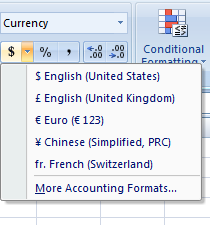Your table should now look something like this:

##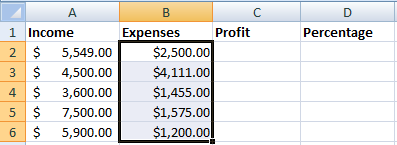What is the Excel formula for percentage?

To get your profit percentage, enter the percentage formula for Excel “=a2-b2” into the c2 Profit cell.

Once you have calculated the profit amount, drag the corner of the cell to include the rest of your table.In order to calculate your profit percentage, enter the following formula into the blank cell under Percentage: = c2 / a2.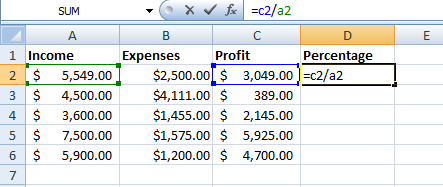Once you have received your profit percentage, drag the corner of the cell to include the rest of your table.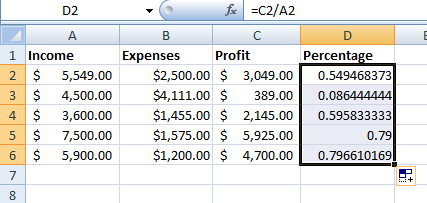You’ll see that you don’t have a profit percentage listed currently, but head over to the General tab up top and select Percentage.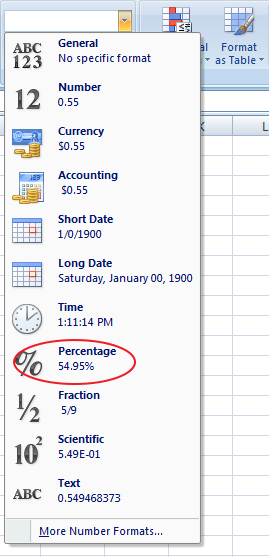Profit percentages will be clearly presented for each cell.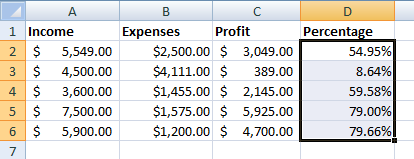You can use the =SUM function as the total profit formula to calculate the total amount of money you have earned. So in this example, you would need to drag column ‘C’: till the last input and at the cell after the last input add = SUM function.

## Setting up a profit calculator excel template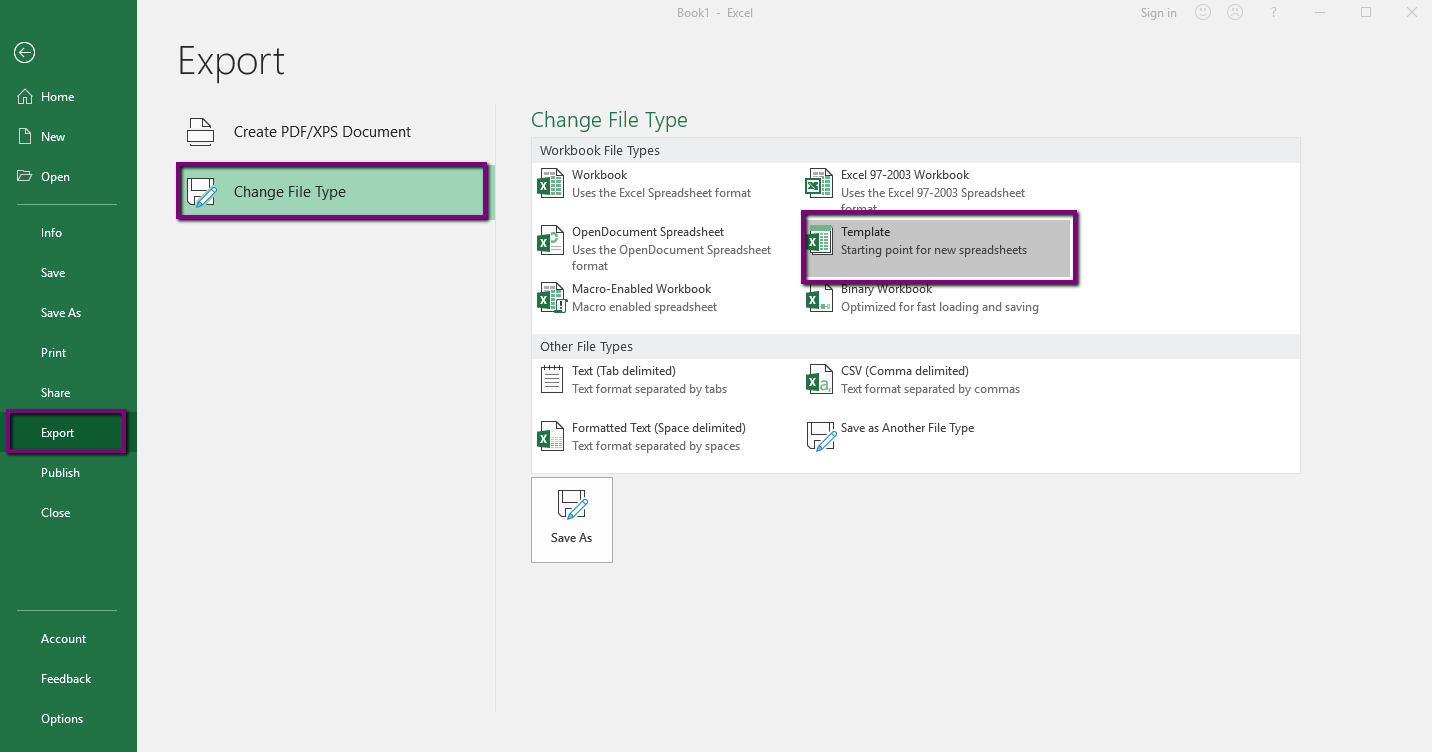So, if you need to calculate profit in Excel quite often, you can save these functions as an template. For instance, you can include the names of the main cells and add profit percentage formula. The next time you need to calculate profit in Excel, you can just load the template.

To create a profit calculator Excel template, first save this documents you have been working with as an Excel workbook. Then open it again and delete the inputs, but make sure the formula is still in function.

Now click on the File menu, Export button and then on Change File Type. From the list of file types choose Templates and click on Save as.